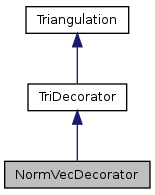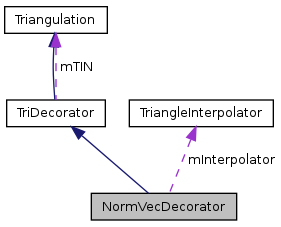QGIS API Documentation  2.0.1-Dufour
NormVecDecorator Class Reference

Decorator class which adds the functionality of estimating normals at the data points. More...

`#include <NormVecDecorator.h>`

Inheritance diagram for NormVecDecorator:[legend]
Collaboration diagram for NormVecDecorator:[legend]

## Public Types

enum  pointState { NORMAL, BREAKLINE, ENDPOINT }
Enumeration for the state of a point. More...

## Public Member Functions

NormVecDecorator ()
NormVecDecorator (Triangulation *tin)
virtual ~NormVecDecorator ()
Adds a point to the triangulation.
bool calcNormal (double x, double y, Vector3D *result)
Calculates the normal at a point on the surface and assigns it to 'result'.
bool calcNormalForPoint (double x, double y, int point, Vector3D *result)
Calculates the normal of a triangle-point for the point with coordinates x and y.
bool calcPoint (double x, double y, Point3D *result)
Calculates x-, y and z-value of the point on the surface and assigns it to 'result'.
virtual void eliminateHorizontalTriangles ()
Eliminates the horizontal triangles by swapping or by insertion of new points.
bool estimateFirstDerivative (int pointno)
Estimates the first derivative a point.
bool estimateFirstDerivatives (QProgressDialog *d=0)
This method adds the functionality of estimating normals at the data points.
Vector3DgetNormal (int n) const
Returns a pointer to the normal vector for the point with the number n.
bool getTriangle (double x, double y, Point3D *p1, Vector3D *v1, Point3D *p2, Vector3D *v2, Point3D *p3, Vector3D *v3)
Finds out, in which triangle a point with coordinates x and y is and assigns the triangle points to p1, p2, p3 and the estimated normals to v1, v2, v3.
bool getTriangle (double x, double y, Point3D *p1, int *ptn1, Vector3D *v1, pointState *state1, Point3D *p2, int *ptn2, Vector3D *v2, pointState *state2, Point3D *p3, int *ptn3, Vector3D *v3, pointState *state3)
This function behaves similar to the one above.
pointState getState (int pointno) const
Returns the state of the point with the number 'pointno'.
void setTriangleInterpolator (TriangleInterpolator *inter)
Sets an interpolator.
virtual bool swapEdge (double x, double y)
Swaps the edge which is closest to the point with x and y coordinates (if this is possible) and forces recalculation of the concerned normals (if alreadyestimated is true)
virtual bool saveAsShapefile (const QString &fileName) const
Saves the triangulation as a (line) shapefile.Public Member Functions inherited from TriDecorator
TriDecorator ()
TriDecorator (Triangulation *t)
virtual ~TriDecorator ()
virtual void addLine (Line3D *line, bool breakline)
Adds an association to a triangulation.
virtual void performConsistencyTest ()
Performs a consistency check, remove this later.
virtual Point3DgetPoint (unsigned int i) const
Returns a pointer to the point with number i.
virtual int getNumberOfPoints () const
Returns the number of points.
bool getTriangle (double x, double y, Point3D *p1, int *n1, Point3D *p2, int *n2, Point3D *p3, int *n3)
bool getTriangle (double x, double y, Point3D *p1, Point3D *p2, Point3D *p3)
Finds out, in which triangle the point with coordinates x and y is and assigns the points at the vertices to 'p1', 'p2' and 'p3.
virtual int getOppositePoint (int p1, int p2)
Returns the number of the point opposite to the triangle points p1, p2 (which have to be on a halfedge)
virtual QList< int > * getSurroundingTriangles (int pointno)
Returns a pointer to a value list with the information of the triangles surrounding (counterclockwise) a point.
virtual double getXMax () const
Returns the largest x-coordinate value of the bounding box.
virtual double getXMin () const
Returns the smallest x-coordinate value of the bounding box.
virtual double getYMax () const
Returns the largest y-coordinate value of the bounding box.
virtual double getYMin () const
Returns the smallest x-coordinate value of the bounding box.
virtual void setForcedCrossBehaviour (Triangulation::forcedCrossBehaviour b)
draws the points, edges and the forced lines
virtual void setEdgeColor (int r, int g, int b)
Sets the color of the normal edges.
virtual void setForcedEdgeColor (int r, int g, int b)
Sets the color of the forced edges.
virtual void setBreakEdgeColor (int r, int g, int b)
Sets the color of the breaklines.
virtual void ruppertRefinement ()
Adds points to make the triangles better shaped (algorithm of ruppert)
virtual bool pointInside (double x, double y)
Returns true, if the point with coordinates x and y is inside the convex hull and false otherwise.
virtual QList< int > * getPointsAroundEdge (double x, double y)
Returns a value list with the numbers of the four points, which would be affected by an edge swap.Public Member Functions inherited from Triangulation
virtual ~Triangulation ()

## Protected Member Functions

void setState (int pointno, pointState s)
Sets the state (BREAKLINE, NORMAL, ENDPOINT) of a point.

## Protected Attributes

Is true, if the normals already have been estimated.
TriangleInterpolatormInterpolator
Association with an interpolator object.
QVector< Vector3D * > * mNormVec
Vector that stores the normals for the points.
QVector< pointState > * mPointState
Vector who stores, it a point is not on a breakline, if it is a normal point of the breakline or if it is an endpoint of a breakline.Protected Attributes inherited from TriDecorator
TriangulationmTIN
Association with a Triangulation object.

## Static Protected Attributes

static const unsigned int mDefaultStorageForNormals = 100000

## Detailed Description

Decorator class which adds the functionality of estimating normals at the data points.

Definition at line 27 of file NormVecDecorator.h.

## Member Enumeration Documentation

Enumeration for the state of a point.

NORMAL means, that the point is not on a breakline, BREAKLINE means that the point is on a breakline (but not an endpoint of it) and ENDPOINT means, that it is an endpoint of a breakline

Enumerator:
 NORMAL BREAKLINE ENDPOINT

Definition at line 31 of file NormVecDecorator.h.

## Constructor & Destructor Documentation

 NormVecDecorator::NormVecDecorator ( )
inline

Definition at line 79 of file NormVecDecorator.h.

 NormVecDecorator::NormVecDecorator ( Triangulation * tin )
inline

Definition at line 84 of file NormVecDecorator.h.

 virtual NormVecDecorator::~NormVecDecorator ( )
virtual

## Member Function Documentation

 int NormVecDecorator::addPoint ( Point3D * p )
virtual

Adds a point to the triangulation.

Reimplemented from TriDecorator.

 bool NormVecDecorator::calcNormal ( double x, double y, Vector3D * result )
virtual

Calculates the normal at a point on the surface and assigns it to 'result'.

Returns true in case of success and false in case of failure

Reimplemented from TriDecorator.

 bool NormVecDecorator::calcNormalForPoint ( double x, double y, int point, Vector3D * result )

Calculates the normal of a triangle-point for the point with coordinates x and y.

This is needed, if a point is on a break line and there is no unique normal stored in 'mNormVec'. Returns false, it something went wrong and true otherwise

 bool NormVecDecorator::calcPoint ( double x, double y, Point3D * result )
virtual

Calculates x-, y and z-value of the point on the surface and assigns it to 'result'.

Returns true in case of success and flase in case of failure

Reimplemented from TriDecorator.

 virtual void NormVecDecorator::eliminateHorizontalTriangles ( )
virtual

Eliminates the horizontal triangles by swapping or by insertion of new points.

If alreadyestimated is true, a re-estimation of the normals will be done

Reimplemented from TriDecorator.

 bool NormVecDecorator::estimateFirstDerivative ( int pointno )

Estimates the first derivative a point.

Return true in case of succes and false otherwise

 bool NormVecDecorator::estimateFirstDerivatives ( QProgressDialog * d = `0` )

This method adds the functionality of estimating normals at the data points.

Return true in the case of success and false otherwise

Referenced by QgsTINInterpolator::initialize().

 Vector3D * NormVecDecorator::getNormal ( int n ) const
inline

Returns a pointer to the normal vector for the point with the number n.

Definition at line 94 of file NormVecDecorator.h.

References mNormVec, and QgsDebugMsg.

 pointState NormVecDecorator::getState ( int pointno ) const

Returns the state of the point with the number 'pointno'.

 bool NormVecDecorator::getTriangle ( double x, double y, Point3D * p1, Vector3D * v1, Point3D * p2, Vector3D * v2, Point3D * p3, Vector3D * v3 )

Finds out, in which triangle a point with coordinates x and y is and assigns the triangle points to p1, p2, p3 and the estimated normals to v1, v2, v3.

The vectors are normaly taken from 'mNormVec', exept if p1, p2 or p3 is a point on a breakline. In this case, the normal is calculated on-the-fly. Returns false, if something went wrong and true otherwise

 bool NormVecDecorator::getTriangle ( double x, double y, Point3D * p1, int * ptn1, Vector3D * v1, pointState * state1, Point3D * p2, int * ptn2, Vector3D * v2, pointState * state2, Point3D * p3, int * ptn3, Vector3D * v3, pointState * state3 )

This function behaves similar to the one above.

Additionally, the numbers of the points are returned (ptn1, ptn2, ptn3) as well as the pointStates of the triangle points (state1, state2, state3)

 virtual bool NormVecDecorator::saveAsShapefile ( const QString & fileName ) const
virtual

Saves the triangulation as a (line) shapefile.

Returns
true in case of success

Implements Triangulation.

 void NormVecDecorator::setState ( int pointno, pointState s )
protected

Sets the state (BREAKLINE, NORMAL, ENDPOINT) of a point.

 void NormVecDecorator::setTriangleInterpolator ( TriangleInterpolator * inter )
inlinevirtual

Sets an interpolator.

Reimplemented from TriDecorator.

Definition at line 89 of file NormVecDecorator.h.

References mInterpolator.

Referenced by QgsTINInterpolator::initialize().

 virtual bool NormVecDecorator::swapEdge ( double x, double y )
virtual

Swaps the edge which is closest to the point with x and y coordinates (if this is possible) and forces recalculation of the concerned normals (if alreadyestimated is true)

Reimplemented from TriDecorator.

## Member Data Documentation

protected

Is true, if the normals already have been estimated.

Definition at line 67 of file NormVecDecorator.h.

Referenced by NormVecDecorator().

 const unsigned int NormVecDecorator::mDefaultStorageForNormals = 100000
staticprotected

Definition at line 68 of file NormVecDecorator.h.

 TriangleInterpolator* NormVecDecorator::mInterpolator
protected

Association with an interpolator object.

Definition at line 70 of file NormVecDecorator.h.

Referenced by setTriangleInterpolator().

 QVector* NormVecDecorator::mNormVec
protected

Vector that stores the normals for the points.

If 'estimateFirstDerivatives()' was called and there is a null pointer, this means, that the triangle point is on a breakline

Definition at line 72 of file NormVecDecorator.h.

Referenced by getNormal().

 QVector* NormVecDecorator::mPointState
protected

Vector who stores, it a point is not on a breakline, if it is a normal point of the breakline or if it is an endpoint of a breakline.

Definition at line 74 of file NormVecDecorator.h.

The documentation for this class was generated from the following file: